Hi I have a unique problem here. People here want to look at the YTD Balance for credits and debits. if i have an account number (Example 1000-1000000) i can use a NL(SUM) to pull it from the db like this. All i need is another column to get the account index and then use it as one of the filters.

=(NL("Sum","GL10110","DEBITAMT","ACTINDX",\$C15,"PERIODID",\$J\$4)

How do I do something similar to this if there are a range of account numbers? I want the sum of all the accounts in that range.? (Example 1000-*******)

Thanks!

#### 7 comments

•Harry Lewis

Hello Sandeep -

Do you want a range of ACTINDX?

If so, set cell C15 to 1000..1000000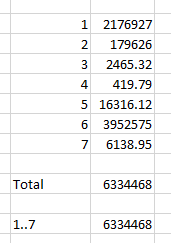•Sandeep Kuntam

Thank you for the reply Harry, well actually I don't want the range of ACTINDX in the way you are telling me. let me try to explain once again.

so I need to get the sum of debits for a range of accounts (Example sum of debits balance for accounts 1000-*******,1010-*******, 3050-******* Etc). In the below table I will show an example of what my current options are.   If i need to get the debit balance of all the accounts that begin with 1000 then I have to first get the ACTINDX for the accounts with =NL("Rows","GL00100","ACTINDX","ACTNUMBR_1",\$F5,"ACTNUMBR_2",\$G5),

Then I need to get the sum of debit balance for that period and that ACTINDX with =(NL("Sum","GL10110","DEBITAMT","ACTINDX",\$E5,"PERIODID",\$H\$3)

so I get the below result.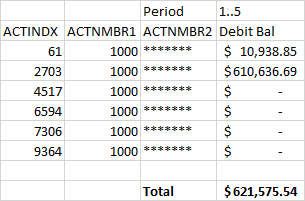Now I dont want to generate this to get the total because there are a lot of account ranges as mentioned before.

What I want to know is, like the GL() where I can just say =GL("Cell","Balance",1000-*******,G\$2,G\$3,,,,,,,,,,,,,,,,,"XYZ","ABCD") it gives me the balance for all the accounts that start with 1000-. is there a way to do that in NL function?

Edited by Sandeep Kuntam
•Harry Lewis

So, you want the total for all accounts that begin with 1000.

That number is also contained in the field ACTNMBR1... correct?

So you should be able to use:

`=NL("Sum","GL10110","DEBITAMT","ACTNMBR1","1000")`

You can, of course, add other filters as needed.

Is there something that I'm missing?

•Sandeep Kuntam

Harry this would be the ideal approach except there is no ACTNMBR1 in GL10110 table hence I have to use the ACTINDX field as a filter and to get the ACTINDX for the correct account I need to get it from GL00100 which is a different table.

•Sandeep Kuntam

Basically I am trying to get the below result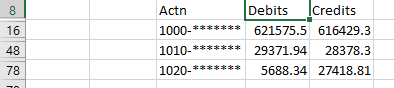but the only right now for me seems to be to write the above mentioned functions and do it this way.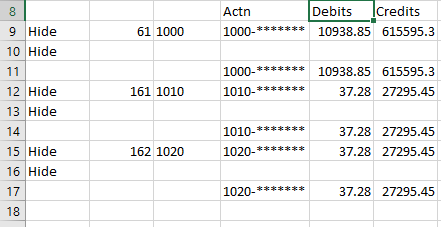trying to see if I am over thinking this and if there is a better and a more efficient way to get the results.

•Harry Lewis

Thank you for the explanation, Sandeep.

Let's look at this example...

The data in my GP database uses three segments.  Let us assume that I only want to consider Segment 1.

From the GL10110 table, I want the sum of the DEBITAMT field where my account number starts with 100 (that is:  Segment 1 is 100).

The account number information I have in the GL10110 table is the ACTINDX field.  However, since this is a key field, I can find it (and other information) elsewhere.  So, what I want to do is find out which ACTINDX values are related to account numbers starting with 100.

I can get this information from the GL00105 table.  That table stores Segment 1 in the field ACTNUMBR_1.

So, I could use NL(Filter) to create a set containing all the ACTINDX where ACTNUMBR_1 is 100

=NL("Filter","GL00105","ACTINDX","ACTNUMBR_1",100)

or, better yet, let's put the 100 in a field and then reference that field: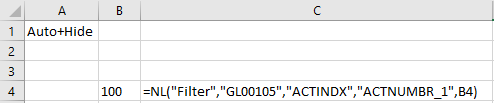Then, I can sum up all the DEBITAMT fields in GL10110 where the ACTINDX is contained in that set: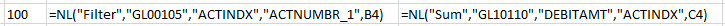If I expand this to include different ACTNUMBR_1 values: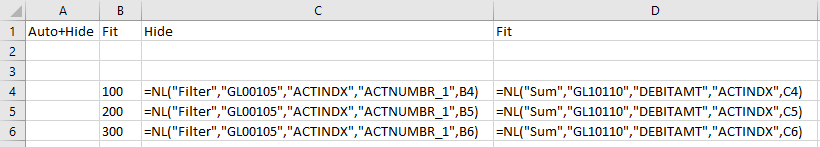I could, of course, also put the NL(Filter) functions directly within my NL(Sum) functions: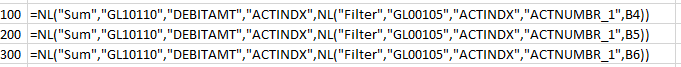I get this when I run my report: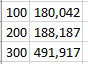Is that more what you are looking for?

•Sandeep Kuntam

Thank you so much Harry.. This is exactly what I was looking for..!! worked perfect..

Please sign in to leave a comment.